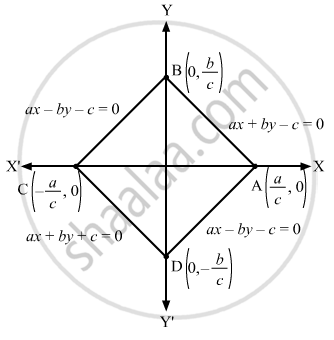Department of Pre-University Education, KarnatakaPUC Karnataka Science Class 11
Advertisement Remove all ads

# The Figure Formed by the Lines Ax ± by ± C = 0 is - Mathematics

MCQ

The figure formed by the lines ax ± by ± c = 0 is

#### Options

• a rectangle

• a square

• a rhombus

•  none of these

Advertisement Remove all ads

#### Solution

a rhombus

The given lines can be written separately in the following manner:
ax + by + c = 0       ... (1)
ax + by − c = 0       ... (2)
ax − by − c = 0       ... (3)
ax − by − c = 0       ... (4)
Graph of the given lines is given below:Clearly,

$\text { AB = BC = CD = DA} = \sqrt{\frac{a^2}{c^2} + \frac{b^2}{c^2}} = \frac{\sqrt{a^2 + b^2}}{\left| c \right|}$

Thus, the region formed by the given lines is ABCD, which is a rhombus.

Is there an error in this question or solution?
Advertisement Remove all ads

#### APPEARS IN

RD Sharma Class 11 Mathematics Textbook
Chapter 23 The straight lines
Q 20 | Page 134
Advertisement Remove all ads

#### Video TutorialsVIEW ALL 

Advertisement Remove all ads
Share
Notifications

View all notifications

Forgot password?